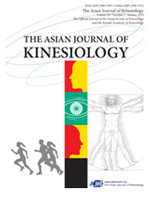상세검색
최근 검색어 전체 삭제
다국어입력
즐겨찾기0KCI등재 학술저널

# 한국인의 체력연령 추정식 개발Develop a Regression Equation of Health-related Physical Fitness Age of Korean Adults

• 등재여부 : KCI등재
• 2012.07
• 81 - 92 (12 pages)

[서론] 본 연구의 목적은 성인과 중년층을 대상으로 체력요인을 활용하여 각 개인의 건강관련체력연령을 추정할 수 있는 추정식을 개발하는 것이다. [방법] 본 연구에 참여한 대상은 수도권에 거주하는 20세에서 65세의 남녀 645명이며, 검사항목은 %bodyfat, BMI, VO2max, PACER, hand grip strength, sit-ups, Sargent jump, sit and reach등 이었다. 주성분분석을 통하여 주성분점수를 산출하고, 주성분점수를 반응변수로 하고 BMI, PACER, sit-ups, Sargent jump, sit and reach를 설명변수로 하는 회귀분석을 실시하여 건강관련체력연령을 추정하기 위한 추정식을 개발하였다. [결과] 개발된 한국인의 건강관련체력연령 추정식은 다음과 같다. 남자 40세 미만 : HPFAma = 45.054+.324X1-.089X2-.142X3-.153X4-.228X5(R2=.954), 남자 40세 이상 : HPFAmm = 75.123+.090X1-.095X2-.382X3-.009X4-.233X5(R2=.909), 여자 40세 미만 : HPFAfa = 49.031+.328X1-.121X2-.423X3-.260X4-.144X5(R2=.913), 여자 40세 이상 : HPFAfm = 76.004+.026X1-.150X2-.651X3-.010X4-.271X5(R2=.865). (X1=BMI, X2=PACER, X3=Sargent jump, X4=sit and reach, X5=sit-ups). [결론]본 연구를 통하여 개발된 건강관련체력연령 회귀추정식은 집단에 관계없이 보편적으로 적용할 수 있는 방식으로 확인되었다.

[INTRODUCTION] The purpose of this study was to develop a regression equation of health-related physical fitness age(HPFA) of Korean adults. The subjects of the study were 646 males and females with ages ranging from 20 to 69 years. The items meas-ured were %bodyfat, VO2max, Hand grip strength, Sit-ups, Sargent jump, Sit and reach, BMI, and PACER. during the analysis, we developed the regression equation against 394 subjects (60% of total subjects; group for equation development) and analyzed a cross validation against 252 subjects (40% of total subjects; group for cross-validation). We grouped subjects of ages 20 to 40 as adults and subjects of ages 40 to 69 as middle-aged adults . [METHOD] Regarding data analysis, first, %bodyfat, VO2max, hand grip strength, sit-up, Sargent jump, sit and reach were set as variables and a principle component score (PCS) was yielded through principal component analysis. Second, sit-up, Sargent jump, sit and reach, BMI, and PACER were set as explanatory variable and the reverse score of PCS as response variable to develop a regression equation through multiple regression analysis. Third, we exe-cuted an independent t-test against the group for cross-validation to verify the difference in chronological age, VO2max, and %bodyfat between below-average HPFA group and above-average HPFA group for cross-validation. [RESULT] The resulting re-gression equations by sex and age were as the following; HPFAma=45.054+.324X1-.089X2-.142X3-.153X4-.228X5(R2=.954) for male adults, HPFAmm=75.123+.090X1-.095X2-.382X3-.009X4-.233X5(R2=.909) for male middle-aged adults, HPFAfa=49.031+.328X1-.121X2-.423X3-.260X4-.144X5(R2=.913) for female adults, and HPFAfm=76.004+.026X1-.150X2-.651X3-.010X4-.271X5(R2=.865) for female mid-dle-aged adults (X1=BMI, X2=PACER, X3=Sargent jump, X4=sit and reach, X5=Sit ups). Regarding the results of the independent t-test against the group for cross-validation, there were statistically significant differences in all groups except %bodyfat of male adults. [CONCLUSION] As a result, the development of regression equation of HPFA through VO2max, BMI, PACER, Sargent jump, Sit-ups, Sit and reach has been proven to be valid.

서론

연구방법

결과

논의

결론 및 제언

참고문헌

로딩중